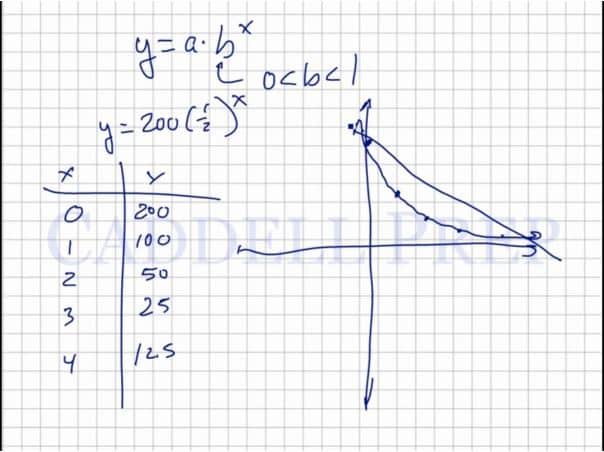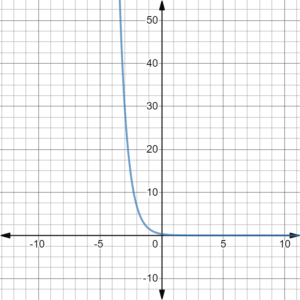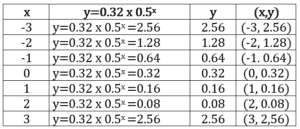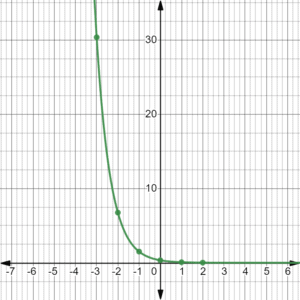In this video, we are going to look at exponential decay. After you finish this lesson, view all of our Algebra 1 lessons and practice problems.$y=a \times (b)^x$
For a graph to demonstrate exponential decay, the base of the exponent, b, must be between 0 and 1. These graphs decrease by a factor, and will not fall into the negatives.
For example:
The graph of$y=200 \times (\frac{1}{2})^x$
curves slowly downward after plotting points as shown in the video, and demonstrates exponential decay. This graph decreases by a factor of$\frac{1}{2}$.Examples of Exponential Decay

Example 1

Does the function$f=\dfrac{1}{3}\cdot \left(\dfrac{2}{9}\right)^x$ model exponential decay?Yes, it shows an exponential decay since the value of$b$ is less than$1$ and the curve is slowly downward.

Example 2

What is the shape of the graph of the function$y=0.32(0.5^x)$?
Now, let’s solve for y and plot the coordinatesThe graph shows a decreasing function. This is an exponential decay.

Video-Lesson Transcript

Let’s go over exponential decay.

Exponential decay is when something decreases by a certain factor over and over again.

You may see this in a chemistry problem or something like that nature.

For example:

We have$200$g of something. It decreases half of the amount so now we have$100$g. Then decrease by half again, we have$50$g. Then decrease by half again, we have$25$g. Then decrease by half again, so we have$12.5$g. And so on.

Here, it decreases by a factor.

It is very different with a linear decay.

Where we have$200$g and when we decrease it by$100$g, we’ll have$100$g. And if we decrease it by$100$g again, we’ll have$0$.

Or let’s decrease at$25$g.

So we have$200$g then decrease to$175$. Then decrease again by$25$, we’ll have$150$.
And so on.

In exponential decay, we keep decreasing and the amount we decrease by also keeps on decreasing.

Whereas in a linear decay, it keeps on decreasing by a certain amount over and over again.

If we think about the linear exponential function we have$y = ab^x$

In exponential decay,$0 \textless b \textless 1$. So its somewhere between$0$ and$1$.

For example:$y = 200 (\dfrac{1}{2})^x$

This one models the one we have before. Whereas$200$ decreases by$\dfrac{1}{2}$ over and over again.

So initially, at time$0$, we have$200$.

When$x = 1$ then$y = 100$,$x = 2$ then$y = 50$,$x = 3$ then$y = 25$,$x = 4$ then$y = 12.5$,
and so on.

Let’s graph this.

But if we have a linear decrease, we’ll have a straight line decrease.

The exponential decay line will not pass through the$x$ axis.

Whereas, the linear decay line will go down into the negatives.